Common Lisp the Language, 2nd EditionNext: Examples Up: Determining the Class Previous: Determining the Class

### 28.1.5.1. Topological SortingTopological sorting proceeds by finding a class C insuch that no other class precedes that element according to the elements in R. The class C is placed first in the result. Remove C from, and remove all pairs of the form (C, D),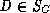, from R. Repeat the process, adding classes with no predecessors to the end of the result. Stop when no element can be found that has no predecessor.

Ifis not empty and the process has stopped, the set R is inconsistent. If every class in the finite set of classes is preceded by another, then R contains a loop. That is, there is a chain of classessuch that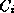precedes, 1 <= i < n, and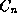precedes.

Sometimes there are several classes fromwith no predecessors. In this case select the one that has a direct subclass rightmost in the class precedence list computed so far.

If there is no such candidate class, R does not generate a partial ordering - the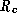,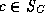, are inconsistent.

In more precise terms, let, m >= 2, be the classes fromwith no predecessors. Let, n >= 1, be the class precedence list constructed so far.is the most specific class, andis the least specific. Let 1 <= j <= n be the largest number such that there exists an i where 1 <= i <= m andis a direct superclass of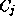;is placed next.

The effect of this rule for selecting from a set of classes with no predecessors is that classes in a simple superclass chain are adjacent in the class precedence list and that classes in each relatively separated subgraph are adjacent in the class precedence list. For example, let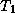and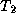be subgraphs whose only element in common is the class J. Suppose that no superclass of J appears in eitheror. Letbe the bottom of; and let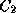be the bottom of. Suppose C is a class whose direct superclasses areandin that order; then the class precedence list for C will start with C and will be followed by all classes inexcept J. All the classes ofwill be next. The class J and its superclasses will appear last.Next: Examples Up: Determining the Class Previous: Determining the Class

AI.Repository@cs.cmu.edu